### Matrices Class 10th Mathematics Tamilnadu Board Solution

##### Question 1.Which one of the following statements is not true?A. A scalar matrix is a square matrixB. A diagonal matrix is a square matrixC. A scalar matrix is a diagonal matrixD. A diagonal matrix is a scalar matrix.Answer:In the above question we see the following terms,Scalar Matrix, Square Matrix, Diagonal MatrixTo answer this question, we have to know the definition of the above terms.Square Matrix:If the rows and columns of the matrices are equal then it will constitute square like structure. So, it is called as square matrix.E.g.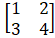Diagonal Matrix:In a square matrix all the elements are zero except the diagonal elements of the matrix. Then that matrix is said to be diagonal matrix.E.g.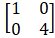Scalar Matrix:In a diagonal matrix, if all the diagonal elements are same then that matrix is said to be a scalar matrix.E.g.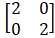Option(A):A scalar matrix should be a square matrix so, it is trueOption(B):Diagonal matrix forms only with the square matrix so, it is trueOption(C):A scalar matrix consists of zero except the diagonal elements so it is true.Option(D):A scalar matrix should comprise of same diagonal elements but in diagonal matrix it may (or) may not contains same diagonal elements. So it is False.Option(D) is not True in the given.Question 2.Matrix A-[aij]mxn is a square matrix ifA. m < nB. m > nC. m = 1D. m = nAnswer:Given that A is a matrix with m and n as their rows and columns respectively.We know that for a square matrix both m and n should be equalSo, m = n is the correct answer.Question 3.If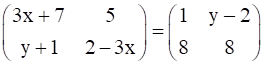then the values of x and y respectively areA. –2, 7B.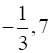C.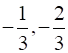D. 2, –7Answer:Given,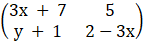=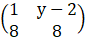If the one matrix is equivalent to other matrix, then their elements should be equal.⇒ 3x + 7 = 1 & 5 = y-23x = -6 ; y = 7x = -2 ; y = 7∴ Option (A) is the answer.Question 4.If A = (1 -2 3) and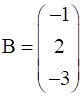then A + BA. (0 0 0)B.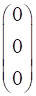C. (-14)D. not definedAnswer:For matrix addition operation their no. of rows and no. of columns should be equal. Otherwise addition is not possible.In the given matrix A has 1 row and 3 columnsBut matrix B has 3 rows and 1 column.Since rows and columns are not equal it is not possible to add.So the answer is (d)Question 5.If a matrix is of order 2 × 3, then the number of elements in the matrix isA. 5B. 6C. 2D. 3Answer:Given that a matrix with order 2x3We know that the no. of elements in the matrix is equal to the product of no. of rows and no. of columnsi.e., No. of elements = No. of rows × No. of columns∴ No. of elements = 2 x 3= 6∴ There will be 6 elements in the given matrixSo, option (B) is the correct answer.Question 6.If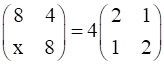then the value of x isA. 1B. 2C.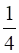D. 4Answer:Given,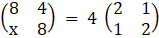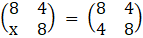Since both the matrix is equal to each other, the elements in it also be equal.By equalizing we will get x = 4So, option (D) is correct answer.Question 7.If A is of order 3 × 4 and B is of order 4 × 3, then the order of BA isA. 3 × 3B. 4 × 4C. 4 × 3D. not definedAnswer:Given matrix orders[A] with 3 x 4 and [B] with 4 x 3We have to find the order of [B].[A]For matrix multiplication the columns of 1st matrix and the rows of 2nd matrix should be equal.E.g. [A]3x2.[B]2x2The order of new matrix formed after multiplication of matrices will be 1st matrix row will be taken as new matrix rows. The no. of columns in 2nd matrix will be taken as new matrix columns.E.g. [A]3x2.[B]2x2 = [AB]3x2In the same way [B]4x3.[A]3x4 = [AB]4x4∴ option (B) is correct answerQuestion 8.If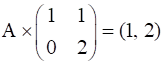, then the order of A isA. 2 × 1B. 2 × 2C. 1 × 2D. 3 × 2Answer:Given,A x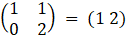Let us consider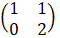as matrix B, it has an order of 2x2Let us consider the resultant matrix as C, it has an order of 1x2For matrix multiplication the columns of 1st matrix and the rows of 2nd matrix should be equal.So, matrix A has got same Columns as Rows of matrix BThe order of new matrix formed after multiplication of matrices will be 1st matrix row will be taken as new matrix rows. The no. of columns in 2nd matrix will be taken as new matrix columnsSo, matrix A has got same Rows as Rows of matrix CSo, Matrix A will consists of 1x2 order∴ option (C) is correct answerQuestion 9.If A and B are square matrices such that AB = I and BA = I, then B isA. Unit matrixB. Null matrixC. Multiplicative inverse matrix of AD. –AAnswer:It is based on a property about multiplication inverse. Only product of a matrix and its own inverse matrix then the resultant matrix will be identity matrix.AB = I and BA = ISo, B is the multiplicative inverse matrix of A∴ option (C) is correct answer.Question 10.If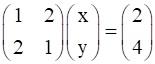, then the values of x and y respectively, areA. 2, 0B. 0, 2C. 0, -2D. 1, 1Answer:Given,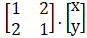=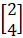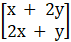=By equating all the elements in the matrixWe will get 2 equationsx + 2y = 2 ⇒ 12x + y = 4 ⇒ 2By solving the both equations we will getx = 2; y = 0∴ option (A) is correct answerQuestion 11.If A =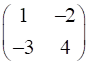and A + B = O, then B isA.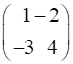B.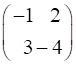C.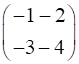D.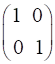Answer:Given thatA + B = O ⇒ B = -A∴ B =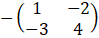B =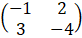∴ option (B) is correct answerQuestion 12.If A =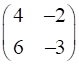, then A2 isA.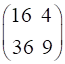B.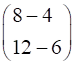C.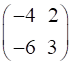D.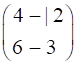Answer:Given A =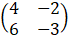A2 = A×A =x=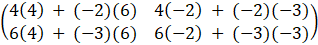=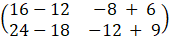=∴ option (D) is correct answerQuestion 13.A is of order m x n and B is of order p x q, addition of A and B is possible only ifA. m = pB. n = qC. n = pD. m = p, n = qAnswer:Given matrix of A is m x n ⇒ [A]mxnMatrix of B is p x q ⇒ [B]pxqWe know that addition is possible is possible only the order is same for both the matricesSo, m = p, n = qSo, option (D) is trueQuestion 14.If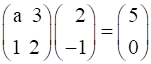, then the value of a isA. 8B. 4C. 2D. 11Answer: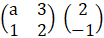=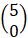By multiplying the matrices, we will get the value of ‘a’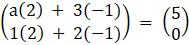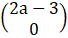=By equating the elements in the matrices2a-3 = 52a = 8a = 4So, option (B) is correct answerQuestion 15.If A =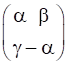is such that A2 = I, thenA. 1 + α2 + βγ = 0B. 1 – α2 + βγ = 0C. 1 – α2 – βγ = 0D. 1 + α2 – βγ = 0Answer:Given A =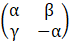A2 = II is the identity matrix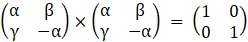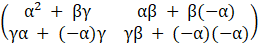=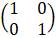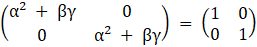By comparing the elements in the matrix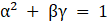⇒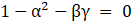∴ Option (C) is correct answer.Question 16.If A = [aij]2x2 and aij = i + j, then A =A.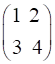B.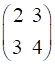C.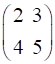D.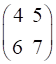Answer:Given A = [aij]2x2 and aij = i + jIt means matrix A with order 2x2 and the elements in is given by aij = i + ja11 = 1 + 1 = 2a12 = 1 + 2 = 3a21 = 2 + 1 = 3a22 = 2 + 2 = 4A =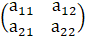=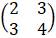∴ Option (B) is correct answer.Question 17.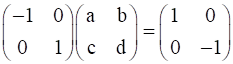, then the values of a, b, c and drespectively areA. -1, 0, 0, - 1B. 1, 0, 0, 1C. -1,0,1,0D. 1, 0, 0, 0Answer:Given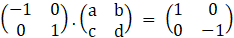By multiplying the matrices, we will get the values of a, b, c & d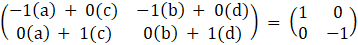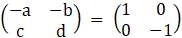By comparing the matrices on both sides-a = 1; -b = 0; c = 0; d = -1∴ Option (A) is correct answer.Question 18.If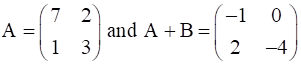, then the matrix B =A.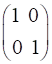B.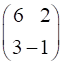C.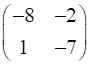D.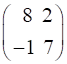Answer:GivenA + B =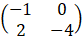; A =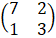B =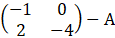B =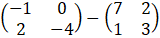=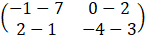=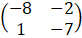∴ Option (C) is correct answer.Question 19.If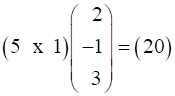, then the value of x isA. 7B. -7C.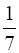D. 0Answer:Given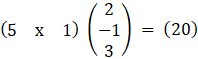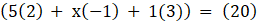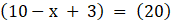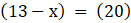By equating the elements13 – x = 20x = -7∴ Option (B) is correct answer.Question 20.Which one of the following is true for any two square matrices A and B of same order?.A. (AB)T = ATBTB. (ATB)T = ATBTC. (AB)T = BAD. (AB)T = BTATAnswer:This is Reversal law for transpose of matrices∴ (AB)T = BTAT∴ Option (D) is correct answer.

PDF FILE TO YOUR EMAIL IMMEDIATELY PURCHASE NOTES & PAPER SOLUTION. @ Rs. 50/- each (GST extra)

HINDI ENTIRE PAPER SOLUTION

MARATHI PAPER SOLUTION

SSC MATHS I PAPER SOLUTION

SSC MATHS II PAPER SOLUTION

SSC SCIENCE I PAPER SOLUTION

SSC SCIENCE II PAPER SOLUTION

SSC ENGLISH PAPER SOLUTION

SSC & HSC ENGLISH WRITING SKILL

HSC ACCOUNTS NOTES

HSC OCM NOTES

HSC ECONOMICS NOTES

HSC SECRETARIAL PRACTICE NOTES

2019 Board Paper Solution

HSC ENGLISH SET A 2019 21st February, 2019

HSC ENGLISH SET B 2019 21st February, 2019

HSC ENGLISH SET C 2019 21st February, 2019

HSC ENGLISH SET D 2019 21st February, 2019

SECRETARIAL PRACTICE (S.P) 2019 25th February, 2019

HSC XII PHYSICS 2019 25th February, 2019

CHEMISTRY XII HSC SOLUTION 27th, February, 2019

OCM PAPER SOLUTION 2019 27th, February, 2019

HSC MATHS PAPER SOLUTION COMMERCE, 2nd March, 2019

HSC MATHS PAPER SOLUTION SCIENCE 2nd, March, 2019

SSC ENGLISH STD 10 5TH MARCH, 2019.

HSC XII ACCOUNTS 2019 6th March, 2019

HSC XII BIOLOGY 2019 6TH March, 2019

HSC XII ECONOMICS 9Th March 2019

SSC Maths I March 2019 Solution 10th Standard11th, March, 2019

SSC MATHS II MARCH 2019 SOLUTION 10TH STD.13th March, 2019

SSC SCIENCE I MARCH 2019 SOLUTION 10TH STD. 15th March, 2019.

SSC SCIENCE II MARCH 2019 SOLUTION 10TH STD. 18th March, 2019.

SSC SOCIAL SCIENCE I MARCH 2019 SOLUTION20th March, 2019

SSC SOCIAL SCIENCE II MARCH 2019 SOLUTION, 22nd March, 2019

XII CBSE - BOARD - MARCH - 2019 ENGLISH - QP + SOLUTIONS, 2nd March, 2019

HSC Maharashtra Board Papers 2020

(Std 12th English Medium)

HSC ECONOMICS MARCH 2020

HSC OCM MARCH 2020

HSC ACCOUNTS MARCH 2020

HSC S.P. MARCH 2020

HSC ENGLISH MARCH 2020

HSC HINDI MARCH 2020

HSC MARATHI MARCH 2020

HSC MATHS MARCH 2020

SSC Maharashtra Board Papers 2020

(Std 10th English Medium)

English MARCH 2020

HindI MARCH 2020

Hindi (Composite) MARCH 2020

Marathi MARCH 2020

Mathematics (Paper 1) MARCH 2020

Mathematics (Paper 2) MARCH 2020

Sanskrit MARCH 2020

Sanskrit (Composite) MARCH 2020

Science (Paper 1) MARCH 2020

Science (Paper 2)

MUST REMEMBER THINGS on the day of Exam

Are you prepared? for English Grammar in Board Exam.

Paper Presentation In Board Exam

How to Score Good Marks in SSC Board Exams

Tips To Score More Than 90% Marks In 12th Board Exam

How to write English exams?

How to prepare for board exam when less time is left

How to memorise what you learn for board exam

No. 1 Simple Hack, you can try out, in preparing for Board Exam

How to Study for CBSE Class 10 Board Exams Subject Wise Tips?

JEE Main 2020 Registration Process – Exam Pattern & Important Dates

NEET UG 2020 Registration Process Exam Pattern & Important Dates

How can One Prepare for two Competitive Exams at the same time?

8 Proven Tips to Handle Anxiety before Exams!